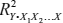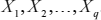This book provides an integrated introduction to multivariate multiple regression analysis (MMR) and multivariate analysis of variance (MANOVA). Beginning with an overview of the univariate general linear model, this volume defines the key steps in analyzing linear model data and introduces multivariate linear model analysis as a generalization of the univariate model. Richard F. Haase focuses on multivariate measures of association for four common multivariate test statistics, presents a flexible method for testing hypotheses on models, and emphasizes the multivariate procedures attributable to Wilks, Pillai, Hotelling, and Roy. The volume concludes with a discussion of canonical correlation analysis that is shown to subsume all the multivariate procedures discussed in previous chapters. The analyses are illustrated throughout the text with three running examples drawing from several disciples, including personnel psychology, anthropology, environmental epidemiology, and neuropsychology.

### Partitioning the SSCP, Measures of Strength of Association, and Test Statistics

In univariate linear model analysis, the value ofis a fundamental statistic for evaluating the extent to which variability in the response variable, Y, is accounted for as a function of the explanatory variables,. The value of ...

•••